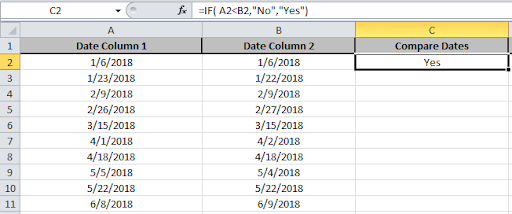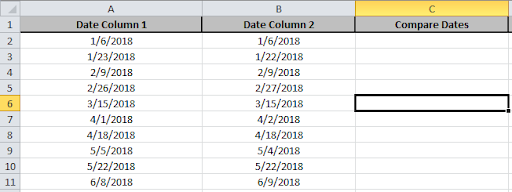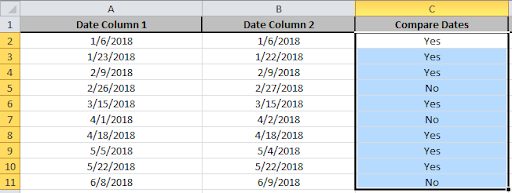# How to Compare Two Dates in Excel?

In this article, we will compare dates using the IF function in Excel 2016. IF function works on the logic test and returns the output on the basis of the test.

IF function tests the condition and returns value either it's True or False.

Syntax of IF function:

 =IF(Logic_test,[value_if_true],[Value_if_false])

Here is an example to show how to compare dates in excel. How do we check if  dates are greater than or equal to, does not equal to, less than, etc in excel

We have two lists named Date Column 1 and Date Column 2. We will compare the two lists using the IF function.Now we will use the IF function in C2 cell
Formula:

 =IF( A2

A2<B2  logic_test to compare dates and returns the values corresponding to it.
“No” value returned if the condition comes True
"Yes" value returned if the condition comes FalseCopy the formula in other cells, select the cells taking the first cell where the formula is already applied, use shortcut key Ctrl+ D.

Your Formula will be pasted using the shortcut and the resulting output will be as shown below.If Dates in Column 2 is greater than Dates in Column 1, No is the response or else then Yes is the response as shown in the above image.

This was an example showing one of the features of the IF function in Excel 2016. Please find more Logic_test function here. If you have any query about the IF function, please do share it in the comment box below. We will help you.

Related Articles:

IF not this or that in Microsoft Excel

Count cells if less than value

IF and Conditional formatting

Popular Articles:

50 Excel Shortcuts to Increase Your Productivity

The VLOOKUP Function in Excel

COUNTIF in Excel 2016

How to Use SUMIF Function in Excel

1.Hi, Can anyone help me out with this. I want a formula which auto calculate according to the month from start date to end date.+

A B C D E F G H I
1 NAME START DATE END DATE NO.OF DAYS AMOUNT PER DAY Jan-13 Feb-13 Mar-13
2 OFFICE 15-Jan-2013 14-Jan-2014 365 80,000.00 219.18 3506.88 6137.04

=IFS(AND(G1<\$B2,G1\$B2,G1<\$C2),(EOMONTH(G1,0))*\$F\$2)
I tried using this formula, but it won't work.

•just tell what each cell contains in this formula `=IFS(AND(G1<\$B2,G1\$B2,G1<\$C2),(EOMONTH(G1,0))*\$F\$2)`.

2.How do you then make Yes turn green and No turn red?

•Using Conditional formatting.

3.I need to compare 2 dates to see if the date an invitation was sent was 21 days or more before the effective date. What formula could I use that will compare those? Thank you!

4.I'm trying to create formula where certain items are due at specific times over several days. I need the date to change once it turns over midnight. I'm assuming this is an IF THEN formula, but i can't seem to make it happen. Any ideas?

5.in the example above, 7/9/2014 (A1) is being compared to A2 the roll below ! is that the intention ?? it looks like we should be comparing A2 and B2 !!!
This is confusing...

6.hi I need to know how to compare three dates and see if they are all the same, also once the comparision is done, cells which are different need to be highlighted in red

•Hi Ayeshah,

Can you please confirm when you say "Cells which are different need to be highlighted in red". Do you mean if 2 cells contain the same date and 1 cell contains the different date then 2 cells should be highlighted instead of 1 who is carrying different date? Please do confirm.

Happy Learning,

7.I want to know the how many days I worked as mentioned dates in 4 work sheet. i.e I want to know how many days I worked in a month depending upon the different work hours mentioned in separate work sheets as dated.Please help me.

•Hi Harsha,

Can you attach sample excel file for the same.

Regards Imran.

8.I typed the date as shown above starting in L2 and entered the formula in N2 "=IF(L2>M2,"NO","YES")" returning the following results: NO NO YES NO YES YES YES NO YES, which is different than the example above. Why would this be?

•You're results are correct with one exception. There is an obvious error in the example given.

You might want to check the data in row 7. 29-Jun-14 is obviously not greater than 26-Jul-14 so the IF result is false and the result should be NO whereas you got YES. All your other data matches my results.

•How to compare if hh:mm are also included with date?

•Hi Bhavana,

Since we know that Excel stores Date & Time as serial numbers where integers part represent the dates and decimal portions represent the time part. So, even if there is time included in any of the criteria, the function will check for the criteria value you will be entering, and will return accordingly.

In case if one criteria does not contain any time value whereas the other contains, excel by default will assume midnight value (00:00:00) for cell which does not have time portion and will return the result accordingly.

Happy Learning!
Team Excel Tip

Terms and Conditions of use

The applications/code on this site are distributed as is and without warranties or liability. In no event shall the owner of the copyrights, or the authors of the applications/code be liable for any loss of profit, any problems or any damage resulting from the use or evaluation of the applications/code.